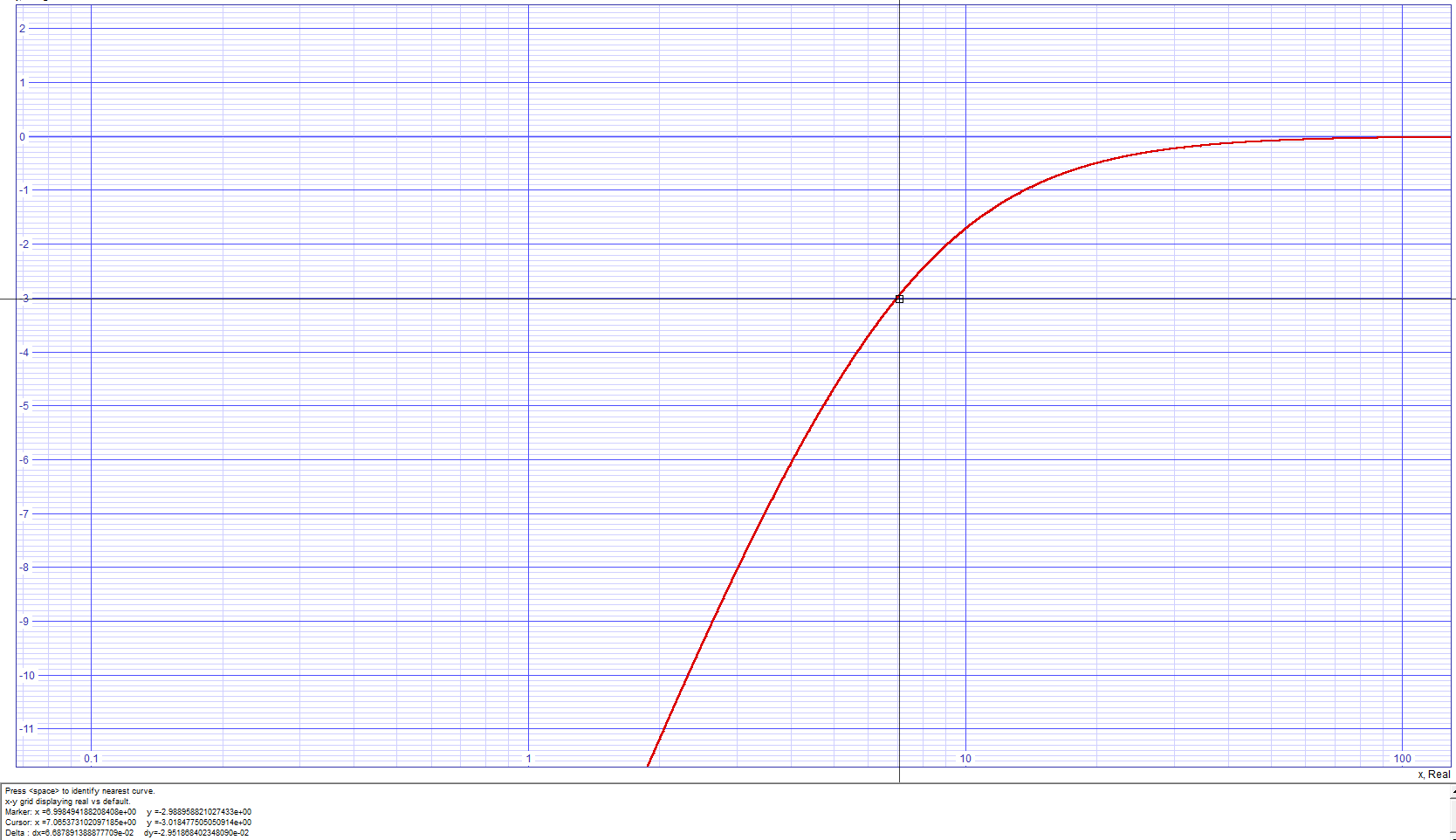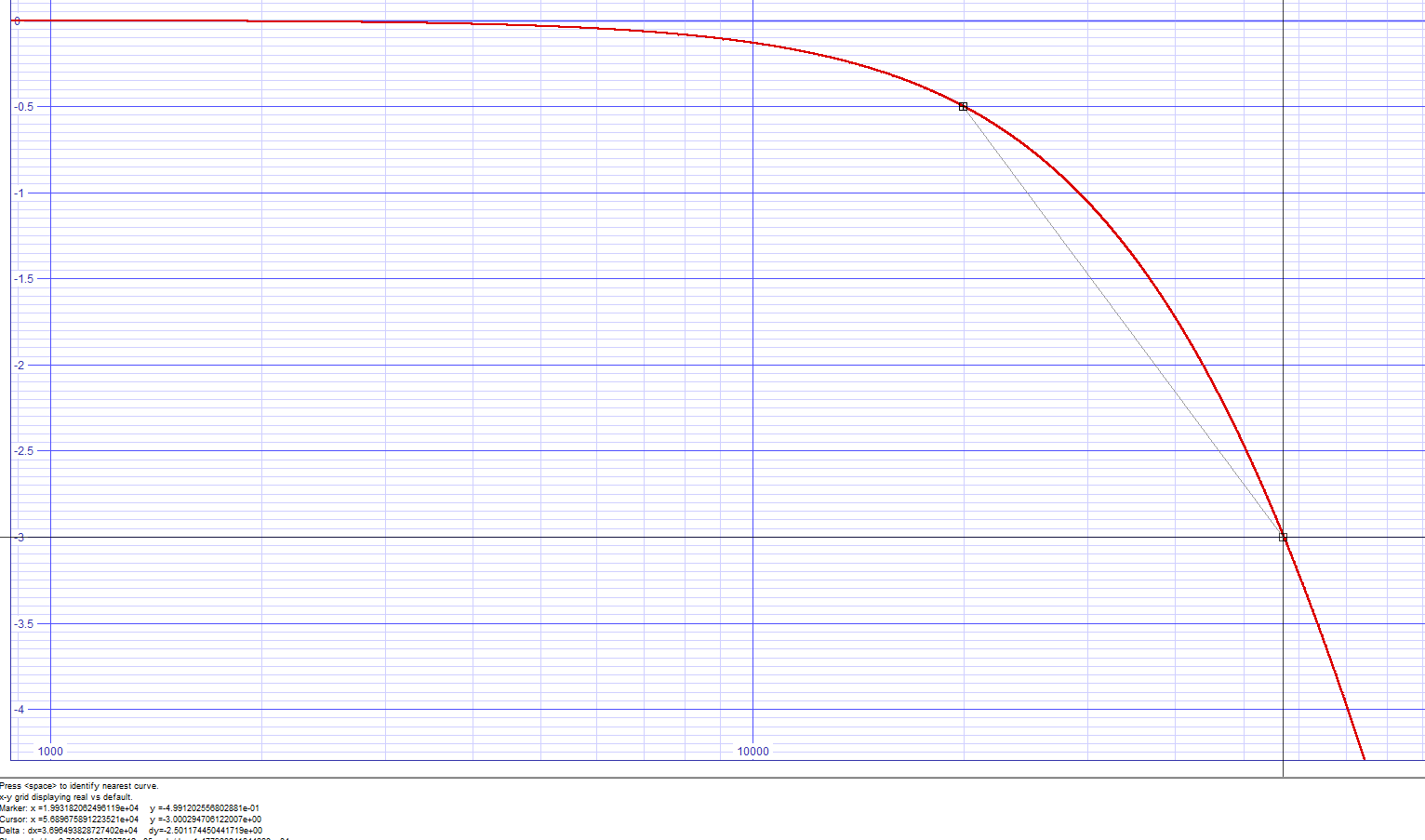# RC Filter Frequency Pole Calculator

There are numerous RC filter pole calculators online and I happily used them for a quick calculations while DIYing. The problem is that these online calculators calculate frequency pole where gain decreases by 3dB, which is  fine, but what if you need to know the frequency of some (first-order) filter while given only the specifications about gain deviation at certain frequency?

### Example

I used to do a lot of audio projects like preamplifiers, compressors, microphones, …  Let’s say we need a standard HiFi frequency response (20 Hz – 20 kHz) of our preamplifier and we want it to be “flat“, meaning that the gain must not deviate for more than 0.5 dB accross whole frequency range until low or high frequency is reached.

Bandwidth (bandpass filter): 20 Hz – 20 kHz
Gain deviation: -0.5 dB

See how to do it or skip this section and use a Python file at the bottom of the page.

Step by step:

• Convert gain from decibels to relative gain.
For example, if filter max. gain is 0 dB, this is a gain of 1.

G(dB) = 20 * log(Gf/G0)    =>    Gf = 10^(-G(dB) / 20) * G0

In our case: -0.5 dB converts to relative gain of 0.9441 (to a reference of 1)

• Get a transfer function of a filter.
For example, our filter is first-order passive RC filter which means that it’s transfer function is:

Gf = G0 / sqrt(1+ (f/fc)2)
for low pass filters (when
f is rising, overall gain is decreasing)

and

Gf = (G0 * (f/fc)) / sqrt(1 + (f/fc))  = G0 / sqrt(1+ (fc/f)2)
for high pass filters (when is lowering , overall gain is decreasing)

Where:
f is our edge frequency (20 or 20kHz),
fc is RC cutoff frequency, where gain decreases by 3 dB
G0 is our reference gain (in our case: 1),
Gf is our desired deviation at chosen frequency f

• Find fc  from the equation above.

fc = f / sqrt( (G0/Gf)-1 )
where
is low pass frequency pole, in our case 20 kHz

and

fc = f * sqrt( (G0/Gf)-1 )
where is high pass frequency pole, in our case 20 Hz

The RC filter frequency poles are we are looking for are at:

1. 6.99 Hz for high pass filter (-0.5 dB at 20 Hz)
2. 57255.50 Hz for low pass filter (-0.5 dB at 20 kHz)
• Calculate resistor or capacitor values.

fc = 1 / (2 * pi * R * C)

Now, sometimes you want small capacitance or you need a specific resitor to meet gain requirements of a filter circuit so you fix one parameter and than calculate the other:

R = 1 / (fc * 2 * pi * C)     OR     C = 1 / (fc * 2 * pi * R)Anyway, it takes time if you are simulating and changing requirements (or if you do it frequently in your spare time :D) to calculate it again and again every time. That is where python script is handy and feel free to download it from here.

## Python script

This is a script to quickly check where pole frequency is and optionally, calculate RC values for this frequency.
Here are the settings:

```########### HIGH PASS FILTER - differentiator
LOW_FREQ = 20 # [Hz] lower frequency (HIGH PASS FILTER)
LOW_FREQ_DEV = 0.5 # [dB] response deviation at LOW frequency

# set either XP_RESISTOR_CALCULATE_CAPACITOR or XP_CAPACITOR_CALCULATE_RESISTOR or none of them
HP_RESISTOR_CALCULATE_CAPACITOR = True
RESISTOR_HP = 10e3 #[ohm]
HP_CAPACITOR_CALCULATE_RESISTOR = False
CAPACITOR_HP = 1e-6 #[F]

########### LOW PASS FILTER - integrator
HIGH_FREQ = 20e3 # [Hz] upper frequency (LOW PASS FILTER)
HIGH_FREQ_DEV = 0.5 # [dB] response deviation at HIGH frequency

# set either XP_RESISTOR_CALCULATE_CAPACITOR or XP_CAPACITOR_CALCULATE_RESISTOR or none of them
LP_RESISTOR_CALCULATE_CAPACITOR = True
RESISTOR_LP = 10e3 # [ohm]
LP_CAPACITOR_CALCULATE_RESISTOR = False
CAPACITOR_LP = 2.2e-6 #[F]

DETAIL = False

```

You can set which component you wish to calculate and which to set in advance. Or don’t use this feature and just calculate wanted frequency.

Output values are printed out like this:

```
High pass frequency pole is at 6.99 Hz for -0.50 dB deviation at 20 Hz.
RC values:
R: 1.00e+04 ohm
C: 2.28e-06 F (calculated)

Low pass frequency pole is at 57255.50 Hz for -0.50 dB deviation at 20000 Hz.
RC values:
R: 1.00e+04 ohm
C: 2.78e-10 F (calculated)
```

Or like this, if DETAIL = True:

```
Detail: Response deviation of -0.50 dB = relative gain of 0.9441 to reference 1.00
Detail: Pole frequency is 6.99 Hz with -0.50 dB deviation at 20 Hz)

High pass frequency pole is at 6.99 Hz for -0.50 dB deviation at 20 Hz.
RC values:
R: 1.00e+04 ohm
C: 2.28e-06 F (calculated)

Detail: Response deviation of -0.50 dB = relative gain of 0.9441 to reference 1.00
Detail: Pole frequency is 57255.50 Hz with -0.50 dB deviation at 20000 Hz)

Low pass frequency pole is at 57255.50 Hz for -0.50 dB deviation at 20000 Hz.
RC values:
R: 1.00e+04 ohm
C: 2.78e-10 F (calculated)

```

Code is commented and you can easily use it as module and use only functions like (example for high pass filter):

```freq = get_pole_freq(LOW_FREQ, LOW_FREQ_DEV, HIGH_PASS)
res_lp = get_res_cap_value(freq, CAPACITOR_HP)
```